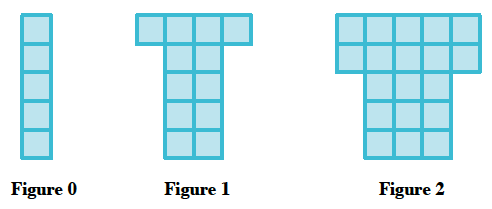### Home > CC4 > Chapter 2 > Lesson 2.1.1 > Problem2-6

2-6.

A tile pattern has five tiles in Figure 0 and adds seven tiles in each new figure. Write a linear equation that represents this pattern. 2-6 HW eTool (Desmos) Homework Help ✎.

Illustrate the problem. Here is one possibility.How many tiles would Figure 3 have?

The figure number is the variable $x$.  The pattern started with $5$ tiles and increased $7$ more for each additional figure. Use this information and the eTool below to find the equation.

Use the eTool below to write the equation of the line that represents the growth of the pattern by completing the table.
Click the link at the right to view full version of the eTool: Int1 2-6 HW eTool.